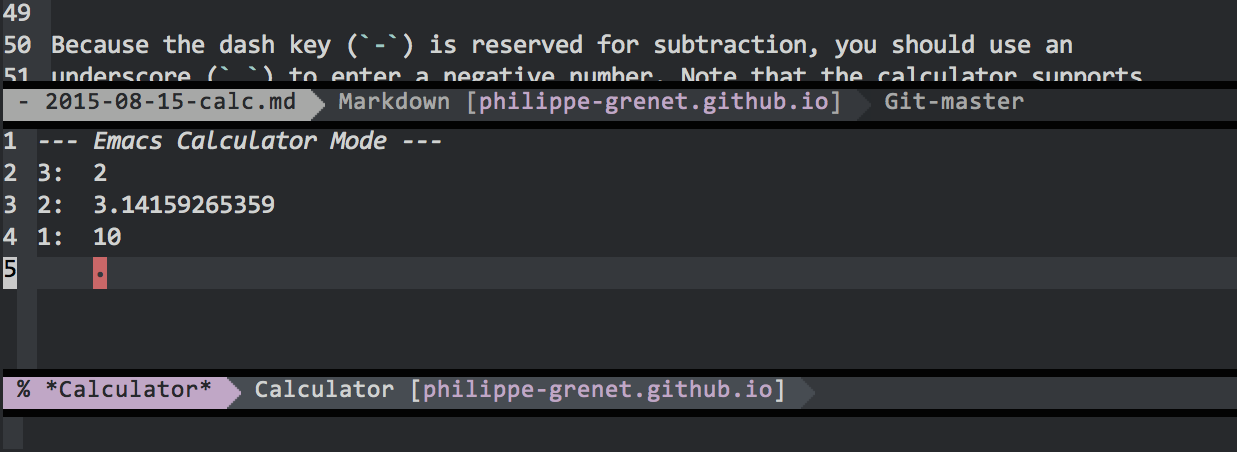# Calc

If you are a programmer, there probably isn’t a day that goes by when you don’t need a calculator, for example for things like base conversion. After all, like Dijkstra said, programming is math.

Emacs includes a calculator, which is actually quite sophisticated. It is capable of both algebraic and symbolic calculations; it has a ton of functions and it can handle matrices or solve equations, if you need that sort of things. It can even create graphs if you have GnuPlot installed on your machine.

Here we will just review the basics. Check out the manual for more. There a nice Info manual embedded in Emacs which you can bring up using ```C-h i g (calc)```.

Start the calculator using `M-x calc`. It will show up at the bottom of the screen. Type `q` to quit. Once the calculator is started, you can hide and redisplay it using `C-x * *`.

### RPN logic

Calc uses the RPN (Reverse Polish Notation) logic. Which is only fair, since Lisp uses the Polish notation! Anyway, if you are a fan of the HP calculators, you will be just at home.

Calc displays a stack of numbers. When you type a number, it accumulates in the stack. When you type an operator such as `+` or `*`, it operates on the last 2 numbers of the stack, and replaces those numbers with the result.

Give it a try: Type `M-x calc`, then the following:

• 2 Enter
• P (no need to press Enter)
• 10 EnterThe `P` inserts π. Now you have 3 numbers in the stack, and you can calculate the circumference of a circle of radius 10 (C = 2 π r). Press `*` once, the stack now shows 2 and 31.4159265359. Press `*` another time and you get a single number which is the result.

Because the dash key (`-`) is reserved for subtraction, you should use an underscore (`_`) to enter a negative number. Note that the calculator supports arbitrarily big numbers. If you want to challenge it, try calculating `2^200` (that’s `2` Enter, `200` Enter, then `^`). The default precision is 12 but you can change it using `p`.

Type `n` to negate the last number, and `TAB` to flip the last 2 numbers. The delete key deletes the last number on the stack.

If you need to use complex numbers, enter them like `(a,b)`. If you need fractions, enter them like `1:3` (1/3). You can also enter an algebraic expression directly by typing `'` (the quote key) then your expression, such as `2 + (10 / 5)`. The result will be put on top of the stack.

Most functions use a single key. For example, type `s` for Sin, `c` for Cos, `t` for Tan, etc. The more complex commands are modal: they start with a prefix key, which leads to a prompt for the subcommand key. If you get lost, type `?` and the mini-buffer will show you what is available.

If you make a mistake, type `U` to undo and `D` to redo. Of course this is Emacs, you have an infinite number of undos and redos. You can also reset the calculator using `C-x * 0`.

### Base conversion

You can enter any number in the format base#number. Example: `16#FF` is immediately converted to 255.

For the reverse, you need to set the output display mode. In this example, ```d r 16``` followed by Enter sets the display to base 16. `d r 2` sets it to binary. Set it to base 10 to get the default behaviour again.